# C Program to Calculate Average Using Arrays

In this tutorial you will learn about the C Program to Calculate Average Using Arrays and its application with practical example.

## C Program to Find Average an Array

In this tutorial, we will learn to create a C program that will average the elements of Array using C programming.

## Prerequisites

Before starting with this tutorial we assume that you are best aware of the following C programming topics:

• Operators in C Programming.
• Basic Input and Output function in C Programming.
• Basic C programming.
• For loop in C Programming.

## Average of the array:-

As we all know array is a collection of similar data type elements. In an array, only one variable is declared which can store multiple values. First will take the number of elements of an array from the user. Then will take the elements from the user for the array. And at last, will total the values and divide them from the number of elements of the array to find the average using C Programming Language.

## Program:-

To find the Average value element from the array

## Output:-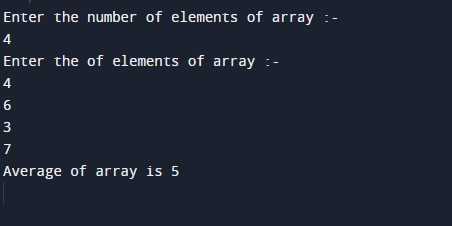In the above program we have first initialized the required variable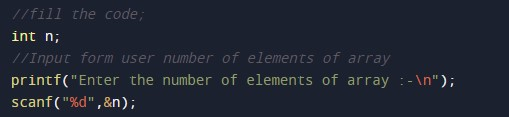• arr = it will hold the elements in an array.
• n = it will hold the number of elements in an array.
• i = it will hold the integer value to control the array.
• sum= it will hold the sum of the array.
• avg= it will hold the average of the array.

Taking input from the user in an array number of elements in the array.Taking input from the user in array elements in the array.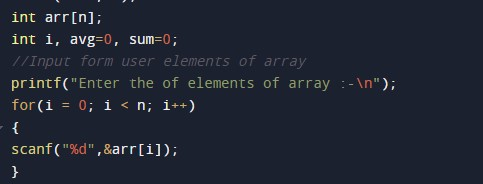Sum of the array.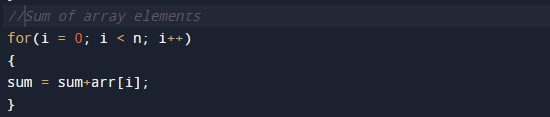Average of Array.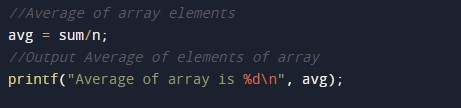In this tutorial we have learn about the C Program to Calculate Average Using Arrays and its application with practical example. I hope you will like this tutorial.